Smartick is an advanced online program that teaches kids math and coding in only 15 min. a day

Jan05

# Discover the Origins of Division and Multiplication

In today’s post, we will explain the origin of the mathematical symbols of division and multiplication.

### The Symbol of Division:

There have been many ways of designating division and we are going to explain the origin of some of the symbols most used and best known by all.

The horizontal bar of fractions, introduced by the Arabs, was first used in Europe by the mathematician Fibonacci in the thirteenth century, although its use did not spread until the sixteenth century.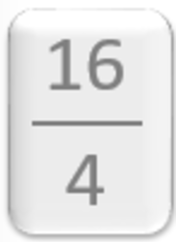The oblique bar, a variant of the horizontal, was introduced by De Morgan in 1845. It was a typographic resource in printed books, in order to be able to write the fraction in a single line. The symbol that is widely used today to express division: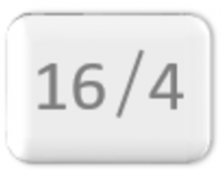Another one of the signs was the parenthesis, although currently it is not used much. To express 21 divided by 3, we would write 21) 3, and place the result of the division to the right after another parenthesis: 21) 3 (7.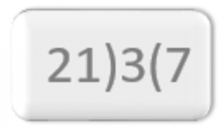This sign is found in the piece Arithmetica integra (1544) by the German mathematician Michael Stiefel.

This same mathematician also used the capital letters M and D to denote multiplication and division in his work Deutsche Arithmetica (1545). Other authors also used a D, including use as an inverted D, such as the French, J. E. Gallimard (1685-1771), and others a fallen d, such as the Portuguese, J. A. da Cuhna (1744-1787).

One of the division symbols still in use is a bar with a point above and below. It was introduced by the Swiss mathematician, Johann Heinrich Rahn, in his work Teutsche Algebra (1659). This division sign is very graphic, to the point that the bar of the fraction is a general norm.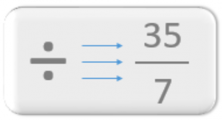This symbol was not very successful in his home country of Switzerland or in Europe. However, it was in both Britain and the United States. In particular, it is still the symbol used on calculators for division.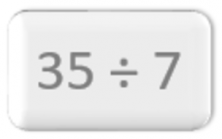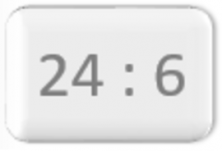The German mathematician, Gottfried W. Leibniz, introduced the two points ( : ), and it is the most widely used symbol nowadays. According to Leibniz, one of the advantages of using this symbol is that the division can be maintained along the same line and maintains the relationship of division with multiplication, for which Leibniz used a point.

As for the gnomon or angle that we use to separate the factors of the division (dividend, divisor, and quotient), there is not much information.

But Boyer, in his History of Mathematics, p.282, says: “The Arabs, and through them later the Europeans, adopted most of their arithmetical artifices from the Hindus, and so it is very probable that the method of ‘long division’ known as the ‘galley method’ by its resemblance to a ship with the sails unfolded, also comes from India.” Apparently, the  “galley method” used an angle similar to the one currently used.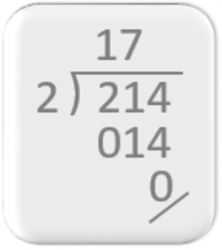### The Symbol of Multiplication:

In the time of the Babylonians, they used an ideogram: “a-du.” In the Bakhshiili manuscript, the oldest manuscript of Indian mathematics, they put one factor side by side and nothing else. The Indian mathematician Bhaskara Acharia (1114-1185) used the word “bhavita” or “bha” just after the factors.

Other mathematicians have used the letter M for multiplication and the letter D for division, as we have said before.In the old days of arithmetic, many algorithms made use of the cross of San Andres to solve division and multiplication products and proportions. It may be for that reason that in 1631, Oughtred, chose this cross as a symbol for multiplication.

It experienced great acceptance, except by the mathematicians Gottfried W. Leibniz and Isaac Newton, who did not feel completely comfortable with the symbol. Leibniz, in 1698, in one of his letters to the mathematician Johann Bernoulli, writes: “I do not like the × symbol as a symbol for multiplication since it can be mistaken for x; … I often simply relate two quantities with a point and indicate multiplication with RS · PQ.”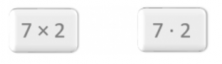For that reason, Leibniz introduced the dot as a symbol of multiplication.

There have been other symbols for multiplication. For example, the Swiss mathematician Johann Rahn, (1622-1676), used the asterisk * in his work Teutsche Algebra (1659). As well as Leibniz, who previously used a fallen C, with the open side down, in his Dissertatio of combinatorial art (1666).

I hope this post about division and multiplication and the symbols that we use to express them has been interesting.